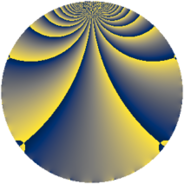# Properties

 Label 2280.2.uLevel $2280$ Weight $2$ Character orbit 2280.u Rep. character $\chi_{2280}(1141,\cdot)$ Character field $\Q$ Dimension $144$ Sturm bound $960$

# Related objects

## Defining parameters

 Level: $$N$$ $$=$$ $$2280 = 2^{3} \cdot 3 \cdot 5 \cdot 19$$ Weight: $$k$$ $$=$$ $$2$$ Character orbit: $$[\chi]$$ $$=$$ 2280.u (of order $$2$$ and degree $$1$$) Character conductor: $$\operatorname{cond}(\chi)$$ $$=$$ $$8$$ Character field: $$\Q$$ Sturm bound: $$960$$

## Dimensions

The following table gives the dimensions of various subspaces of $$M_{2}(2280, [\chi])$$.

Total New Old
Modular forms 488 144 344
Cusp forms 472 144 328
Eisenstein series 16 0 16

## Trace form

 $$144q - 8q^{2} + 8q^{4} - 16q^{7} - 8q^{8} - 144q^{9} + O(q^{10})$$ $$144q - 8q^{2} + 8q^{4} - 16q^{7} - 8q^{8} - 144q^{9} + 24q^{14} - 8q^{16} + 8q^{18} + 16q^{20} + 24q^{22} + 32q^{23} - 24q^{24} - 144q^{25} - 16q^{26} + 16q^{28} - 8q^{32} - 40q^{34} - 8q^{36} - 24q^{42} + 16q^{44} - 16q^{46} + 144q^{49} + 8q^{50} + 24q^{52} - 32q^{55} - 8q^{56} + 24q^{62} + 16q^{63} - 40q^{64} + 24q^{68} + 16q^{70} + 32q^{71} + 8q^{72} - 24q^{74} + 24q^{78} - 32q^{79} + 144q^{81} - 72q^{82} + 48q^{84} + 72q^{86} - 48q^{87} - 104q^{88} + 24q^{92} + 8q^{98} + O(q^{100})$$

## Decomposition of $$S_{2}^{\mathrm{new}}(2280, [\chi])$$ into newform subspaces

The newforms in this space have not yet been added to the LMFDB.

## Decomposition of $$S_{2}^{\mathrm{old}}(2280, [\chi])$$ into lower level spaces

$$S_{2}^{\mathrm{old}}(2280, [\chi]) \cong$$ $$S_{2}^{\mathrm{new}}(24, [\chi])$$$$^{\oplus 4}$$$$\oplus$$$$S_{2}^{\mathrm{new}}(40, [\chi])$$$$^{\oplus 4}$$$$\oplus$$$$S_{2}^{\mathrm{new}}(120, [\chi])$$$$^{\oplus 2}$$$$\oplus$$$$S_{2}^{\mathrm{new}}(152, [\chi])$$$$^{\oplus 4}$$$$\oplus$$$$S_{2}^{\mathrm{new}}(456, [\chi])$$$$^{\oplus 2}$$$$\oplus$$$$S_{2}^{\mathrm{new}}(760, [\chi])$$$$^{\oplus 2}$$